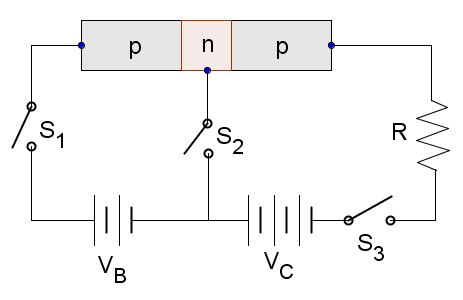# p-n-p transistor and ResistanceThe above is the circuit diagram of a p-n-p type transistor linked with power equipment, resistance $R$, and switches $S_1, S_2$ and $S_3$. Which of the following statements are correct?

a) If switches $S_1$ and $S_3$ are closed and $S_2$ is open, then the electricity flows into resistance $R$.

b) If switch $S_1$ is open and $S_2$ and $S_3$ are closed, then the electricity flows into resistance $R$.

c) If all $3$ switches $S_1, S_2$ and $S_3$ are closed, then the electricity flowing into the collector ($V_C$) is larger than the electricity flowing into the base ($V_B$).

×